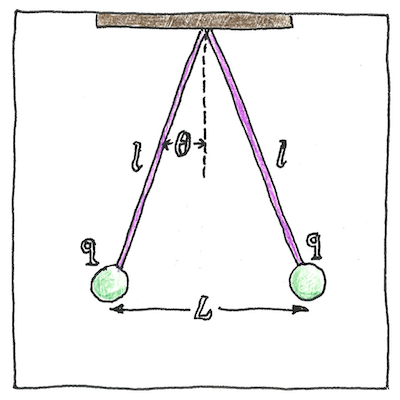# 1785

## The book of science

Tom Sharp

 Charles-Augustin de Coulomb electromagnetism

## Coulomb’s law

• The force between two objects
• that are electrostatically charged
• is directly proportional
• to the magnitude of the charges multiplied together
• and inversely proportional
• to the square of the distance between the objects.
• This law applies
• to any two charged objects with the same polarity,
• to metal spheres
• and to elementary particles.

## History

• Daniel Bernoulli and Alessandro Volta,
• who measured the attractions of capacitor plates,
• and Franz Ulrich Theodor Aepinus
• all supposed the inverse-square law.
• Joseph Priestley,
• who experimented with charged spheres,
• proposed the inverse-square law.
• John Robison
• also measured the force between two charged spheres,
• and announced the inverse-square law.
• Henry Cavendish,
• who later weighed the world with his torsion balance,
• discovered the relationship
• to both distance and amount of charge
• but did not publish.
• Finally, Charles-Augustin de Coulomb,
• after whom the law is named,
• proved the inverse-square law with a torsion balance
• and published the general law.

## Analogies

• Analogous to Isaac Newton’s law
• of universal gravitation
• is Coulomb’s law
• of electrostatic attraction and repulsion,
• which can be used to derive Gauss’s law
• for electric fields,
• which is analogous to Ampère’s circuital law
• for magnetic fields,
• which reminds me of the analogy
• between atoms and solar systems.
• Natural laws are only analogies
• for conditions in the real world
• but we are creatures of analogy;
• all our experiences are analogies.

Coulomb’s law was a significant step in understanding electromagnetic forces. With the work of Volta, Ørsted, Ampère, and Faraday, it led to the invention of electric generators and motors.